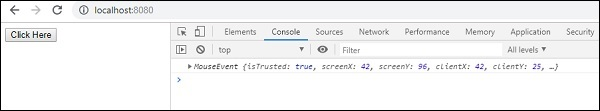# RxJS - Filtering Operator sample

This operator will give the most recent value from the source Observable, and the output will depend upon the argument passed to it emits.

## Syntax

```sample(notifier: Observable): Observable
```

## Parameters

notifier − The argument notifier is an Observable which will decide the output to be picked.

## Return value

It returns an observable, based on values emitted by the source observable.

## Example

```import { fromEvent, interval } from 'rxjs';
import { sample} from 'rxjs/operators';

let btn = document.getElementById("btnclick");
let btn_clicks = fromEvent(btn, 'click');
let case1 = btn_clicks.pipe(sample(interval(4000)));
case1.subscribe(x => console.log(x));
```

The sample() operator is given interval(4000) so the click event will get emitted when the interval of 4seconds is done.

## Output